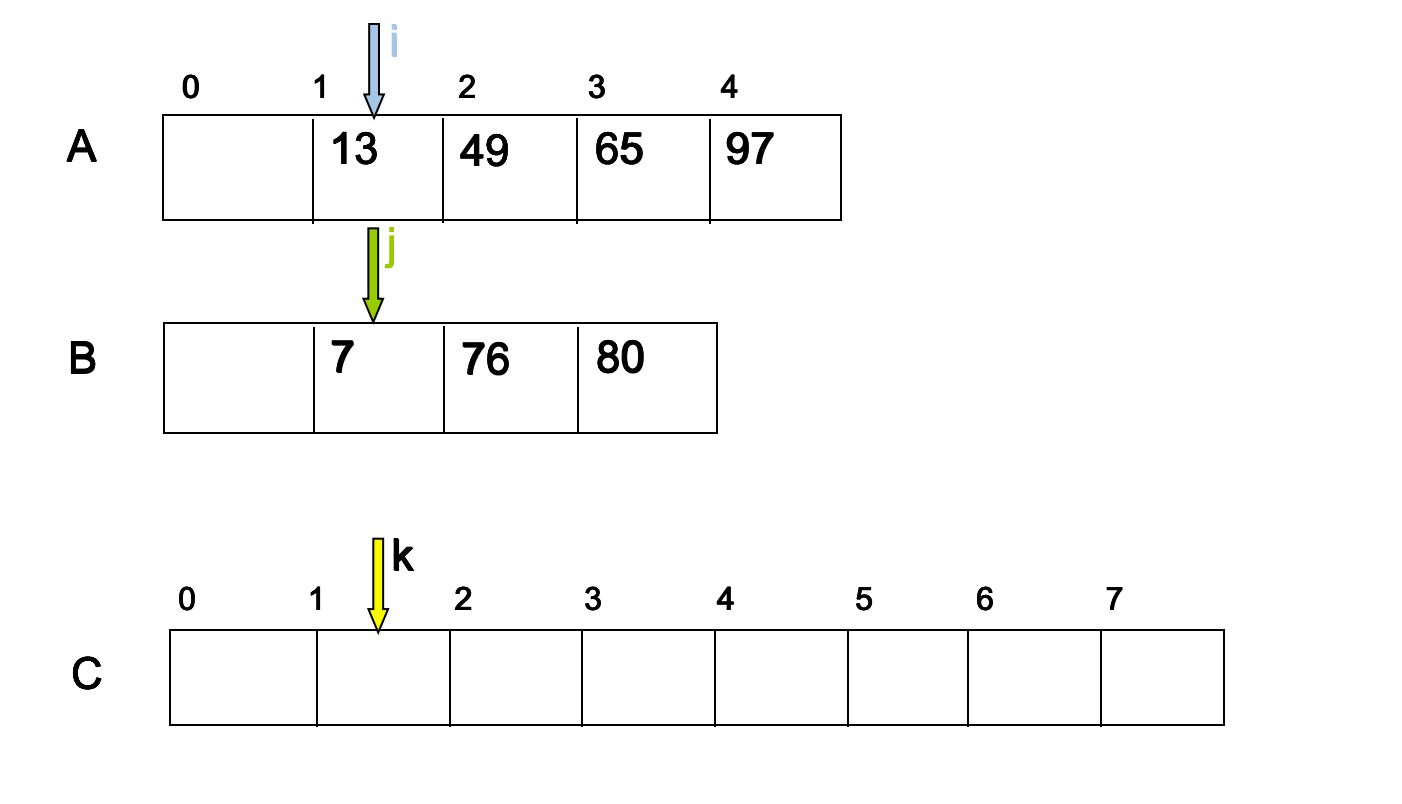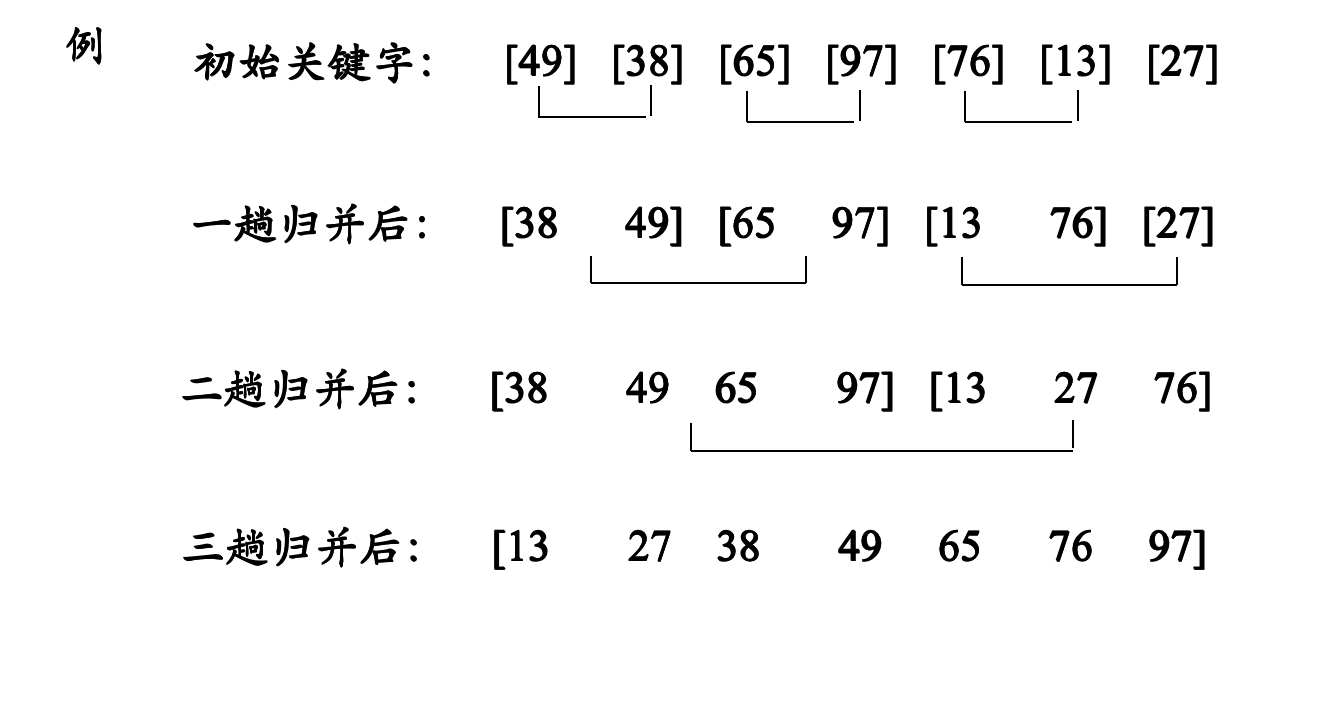# 二、排序过程

1. 初始序列看成n个有序子序列，每个子序列长度为1
2. 两两合并，得到（n/2向下取整数）个长度为2或1的有序子序列
3. 再两两合并，重复直至得到一个长度为n的有序序列为止

1.将一个数组分成两个数组，分别对两个数组进行排序。

2.循环第一步，直到划分出来的“小数组”只包含一个元素，只有一个元素的数组默认为已经排好序。

1.将两个有序的数组合并到一个大的数组中。

2.从最小的只包含一个元素的数组开始两两合并。此时，合并好的数组也是有序的。

## 1.将两个顺序表合并成一个有序表int [] mergeSort(int a[] , int b[],){
int c[a.length + b.length] ;
int i = 0;
int j = 0;
int k = 0;
while (i < a.length && j < b.length){
if ( a[i] < b [j]){
c[k ++] = a[i];
i ++;
}else{

c[k ++] = b[j];
j++;
}
}
while ( i < a.length ){
c[k] = a [i];
i ++;
k ++;
}
while ( j <b .length ){
c[k] = a [j];
j ++;
k ++;
}
return c;
}



## 2.过程## 四.完整代码

public class MergeSort {

public static int[] sort(int[] nums, int low, int high) {
int mid = (low + high) / 2;
if (low < high) {
// 左边
sort(nums, low, mid);
// 右边
sort(nums, mid + 1, high);
// 左右归并
merge(nums, low, mid, high);

}
return nums;
}

public static void merge(int[] nums, int low, int mid, int high) {
int[] temp = new int[high - low + 1];
int i = low;// 左指针
int j = mid + 1;// 右指针
int k = 0;

// 把较小的数先移到新数组中
while (i <= mid && j <= high) {
if (nums[i] < nums[j]) {
temp[k++] = nums[i++];

} else {
temp[k++] = nums[j++];

}

}
// 把左边剩余的数移入数组
while (i <= mid) {
temp[k++] = nums[i++];

}

// 把右边边剩余的数移入数组
while (j <= high) {
temp[k++] = nums[j++];

}

// 把新数组中的数覆盖nums数组
for (int k2 = 0; k2 < temp.length; k2++) {
nums[k2 + low] = temp[k2];

}

}

}



https://www.cnblogs.com/horizonice/p/4102553.html
《数据结构》 严蔚敏
《算法导论》2.1章节

posted @ 2018-12-12 00:01  辰砂tj  阅读(1569)  评论(1编辑  收藏